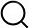# Also in the Article

Relabeling operators

Constructing graphs from genetic encodings
Sci Rep, Jun 24, 2021;

Procedure

In this manuscript we have utilized the $(01X)O(10X)$ formalism to introduce links into a network. In this section we explore how such wiring rules can be expressed in matrix form, thereby providing a mathematical foundation to the $O$ “operator.” In order to do so, we must consider a transformation to a “label space,” where L represents a neuron’s participation in sets (i.e. (01X), or other bit combinations), each its own label. For instance, the nodes 010 and 011 would participate in the label (01X). The importance of labels for mathematical inference over combinatorial compatibility rules has been previously previously discussed in10.

We propose representing a single label transformation as a single-layer neural network, $l=f(WX+d)$, where X is the binary bx1 vector of a single neuron’s expression of b bits, W is a 1xb vector representing a bit’s contribution to a label, d is a normalization term, and $f(b+1)=1$, but is otherwise 0. Specifically, $Wa=1$ if bit a is necessary for a label, $Wa=-1$ if expression of bit a inhibits label participation, and $Wa=0$ if the value of bit a has no effect on label participation (is X: can be expressed or non-expressed). Returning to the example of the set (01X), its W matrix would consist of $[-1,1,0]$. The scalar d is a normalization term, such that $bexp+d=b+1$, where $bexp$ is the number of bits required to be expressed for the rule, and $b+1$ is chosen such that with binary expression and the − 1, 0, + 1 of W, a neuron has no chance of being mistakenly assigned to a label. Thus, for the set (01X), $d=3$. In this way, nodes with identity 010 and 011 will all come out to $l=1$ after $l=f([-1,1,0]∗X+3)$ (Given that $(0∗-1+1∗1+0∗0)+3=4$, $(0∗-1+1∗1+0∗0)+3=4$, and $4=b+1$, so $f(4)=1$).

When expanding the formalism to account for all l labels, $L=f(WX+D)T$, where L is a NxL matrix, W is a Lxb matrix of all labeling rules, X is the bxN matrix of all cells’ expression patterns, and D is a LxN matrix of biases (such that columns are identical). We can now define an O matrix of size LxL which defines the label interactions that lead to connectivity formation. For instance, given $(01X)O(10X)$, if the two labels (01X) and (10X) were indexed at $Li$ and $Lj$, we have $Oij$ = 1.

Combining all derived relations, we can generate the NxN connectivity matrix B by

In previous work, the relation had been expressed as $B=XOXT$10.

Q&A